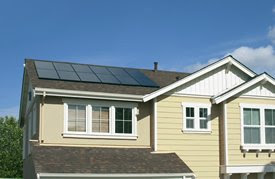## Thursday, December 11, 2008

### Calculating Your Solar Power RequirementsOne of the most important thing you have to figure out when planning a solar power installation is the size of the solar system you’ll need.

First of all you need to calculate how much power you use on a daily basis. Your electric bill will be of help for this or you can make your own calculation. The power consumption of appliances is given in Watts (eg 21" fluorescent light, 13W). To calculate the energy you will use over time, you have to multiply the power consumption by the hours of use.

The 13W light fitting, on for 2 hours, will take 13 x 2 = 26WH.

Repeat this for all the appliances you wish to use, then add the results to obtain your daily power consumption.

You have to determinate also how much energy your battery can store. Battery capacity is measured in Ampere Hours (Ah). Convert this to Watt Hours by multiplying the Ah figure by the battery voltage: for a 20Ah, 12V battery the Watt Hours figure is 20 x 12 = 240WH.

The more energy you take, the faster the battery discharges. Keep in mind, however, that deep cycle batteries which are usually used in solar power systems, should not be discharged beyond 50% of their capacity. i.e. 50 % capacity remaining. Discharging beyond this level will significantly reduce the life of the batteries.

To calculate the energy your solar panel can supply to the battery, multiply Watts by the hours exposed to sunshine, then multiply the result by 0.85 (factor to allow for normal system losses).

For example, the Solar 40W panel in 4 hours of sunshine, 40 x 4 x 0.85= 136WH. This is the amount of energy the Solar panel can supply to the battery.

On the basis of all these calculations you'll determinate the size and number of solar panels you'll need, the number of batteries, power inverter size, etc.